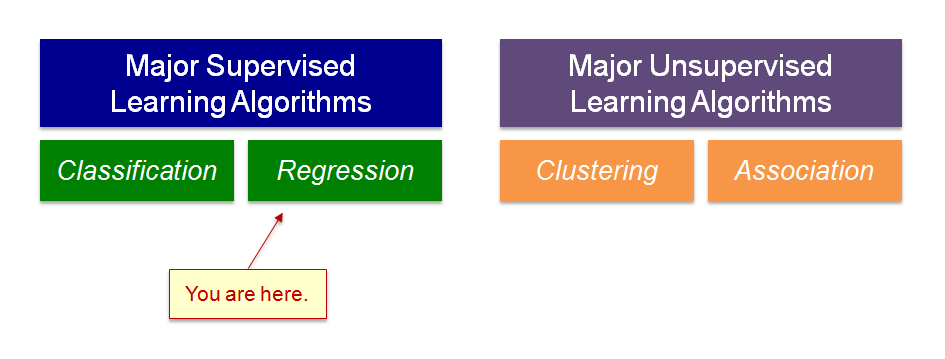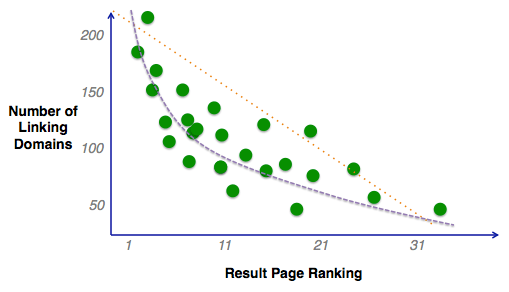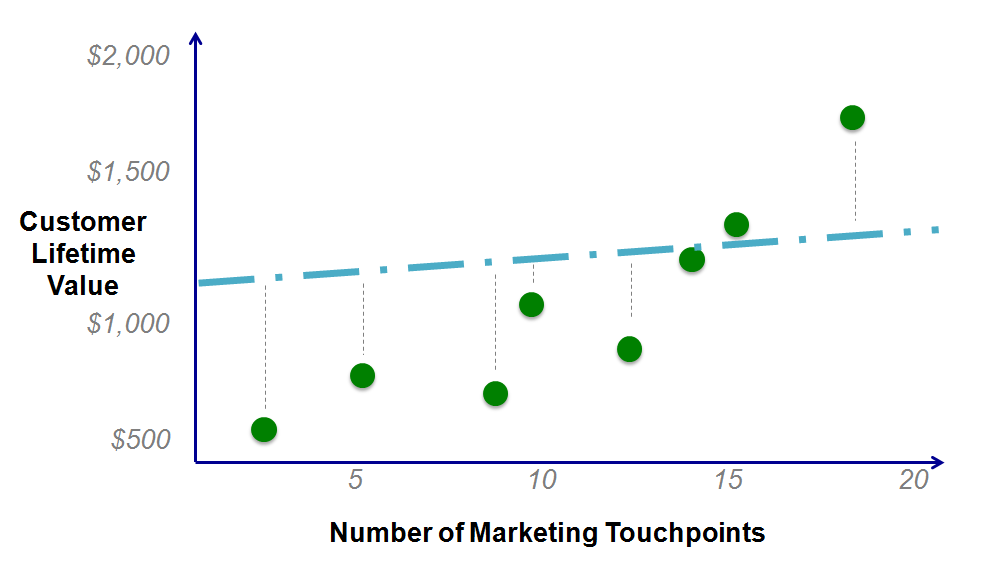# Univariate Linear Regression Concepts

Howdy, machine learning compatriots! Welcome back to our foray into getting started with machine learning. Previously, we covered some core machine learning concepts, namely supervised machine learning algorithms and unsupervised / deep learning. (For the full series to date, here’s our Machine Learning for Beginners page.)   Today we’re learning the concepts behind supervised machine […]

Howdy, machine learning compatriots! Welcome back to our foray into getting started with machine learning. Previously, we covered some core machine learning concepts, namely supervised machine learning algorithms and unsupervised / deep learning. (For the full series to date, here’s our Machine Learning for Beginners page.)

Today we’re learning the concepts behind supervised machine learning algorithms. Specifically, we examine univariate (one variable) linear regression. Univariate linear regression is the beginner’s playpen in supervised machine learning problems. We endeavor to understand the “footwork” behind the flashy name, without going too far into the linear algebra weeds.

## Quick Recap: Supervised Machine Learning Problems

If you’re just dropping into the series, we’ll quickly set today’s stage. Univariate linear regression falls under the category of regression algorithms, withing supervised learning machine learning problems.• Supervised learning: we provide the algorithm with pre-cleaned, pre-labeled data. The algorithm learns off the data we provide to classify or predict new data.
• Regression: making a line of best fit.

When we first covered supervised machine learning concepts, regression was shown to make a line of best fit from existing data, so we could predict new data points. Below, we first used the example of an SEO team predicting how many unique linking domains a page would need to achieve a certain rank. (A supervised learning problem, using a regression algorithm for future predictions.)Important note: our graphic above is similar to linear, but is not quite, linear regression. Details, details. At any rate, this should take us in nicely to examining the inner workings of univariate linear regression.

## A High Level Look at the Regression Problem Process

If I’m being brutally honest, the process of translating machine learning education to public-facing blog posts has been my toughest effort to date. In other words, I try to make my posts easy to follow, like dummy notes I’m taking as I learn.

That being said, 2 weeks into a machine learning course, and the content has already gone off the wheels, deep into linear algebra and so forth. So, instead of going into the weeds for publication, I’m trying to keep it snackable (buzzword bingo, drink!) and down to earth.

Let’s settle in slowly on the regression problem process. Also illustrated below, we need a few key steps:

1. A cleaned training data set with correct labels
2. A program (such as Matlab or Octave) with functionality and access to an appropriate univariate linear regression algorithm
3. A hypothesis and prediction of new valuesLet’s peel back a layer and go slightly deeper. Since cleaning and correctly labeling training data is largely dependent on you & your domain, we’re skipping that step. ¯_(ツ)_/¯

Instead, let’s look closer at the algorithm & hypothesis portions! Our first stop is something called the cost function.

## The Cost Function in Linear Regression Learning Problems: Squared Error

Before we jump into cost function, let’s turn over a new leaf in visual examples. Instead of our SEO example, let’s look at a problem that could be more linear-friendly. Below, let’s assume we have some data on a customer’s lifetime value plotted against the number of marketing touchpoints they’ve interacted with.Okay, with the housekeeping complete, let’s remember our goal for linear regression: find the line of best fit.

Let’s also tie this back to the real world. Perhaps we’re a marketing director or VP of marketing needing to convey the ideal number of marketing touchpoints to the CMO and CEO. Doing so could help guide budgeting, channel mix, and planning questions.

How do we find a line of best fit? Through linear algebra and programming, we can objectively determine the best fit by testing hypotheses and measure each hypothesis line against the actual data points for closeness of fit.Being frank, the material up to this point is pretty humdrum. However, when we start making hypotheses such as the above, things get interesting. The program “makes a guess” as to the line of best fit, perhaps like the illustration above. I’m no “eggspert”, but that doesn’t look like a great line of fit.

But have no fear dear reader, math/science comes to the rescue. The next portion of the algorithm calculates the distance (cost function / squared error) from the training data to the predicted line of best fit in a process called squared error function. When you plot the hypothesis against the squared error sum, you may get a distribution something like the below.Bare with me. Let’s say we plotted:

1. Our illustrated hypothesis (teal plus sign)
2. Other attempted hypotheses (tan x’s), and,
3. The best fit hypothesis (green outlined star)

This renders a convex parabolic distribution. To get the line of best fit, we want to get as low on the X axis as possible. (Known as the global minimum.) The further magic in machine learning is how we move from a lame hypothesis (teal plus sign) to solution (green outlined star). Now, meet a technique called gradient descent. Sidebar: if we’re being more mathematical and technical about it, this really plots to a 3D conic distribution, but the above explanation should suffice for now if we’re not getting bogged down in the math.

## Parameter Learning & Gradient Descent

Gradient descent is the iterative mathematical process of working our way down the squared error plot from a lousy hypothesis to a line of best fit. Again, we’re not delving into calculations and derivatives – there’s a TON of math that goes behind this material.

Gradient descent systematically tests increments of hypotheses against a specified learning rate. The learning rate is essentially the magnitude or speed with which you which try to move along the convex function down to zero.## Wrap Up

Did I mention this is one of the toughest posts for me to date? The other contender is my DIY Alexa Raspberry Pi article. It’s now 3:20 a.m. on a Saturday night/Sunday morning as I type this conclusion. (Insert horror emoji.)

So, if we were to break down all of the above into a short bulleted list:

1. Univariate linear regression takes sample data to make a line of best fit
2. “Best fit” is objectively measured by a squared error function, or the summed distances of the hypothesis line from the actual data points
3. The hypothesis and squared error function plot roughly a convex parabolic graph
4. Gradient descent is an algorithm that systematically reduces the squared error hypothesis, guided in part by the learning rate
5. The gradient descent iteratively seeks the global minimum on the convex function, AKA the line of best fit
6. The line of best fit is determined, (and Teh Lurd of Teh Rings finishes on your second monitor to Herb Alpert’s Spanish Flea.)

Next up, we’ll be installing some machine learning software (Matlab & Octave) and diving into multivariate regression. Look after each other.